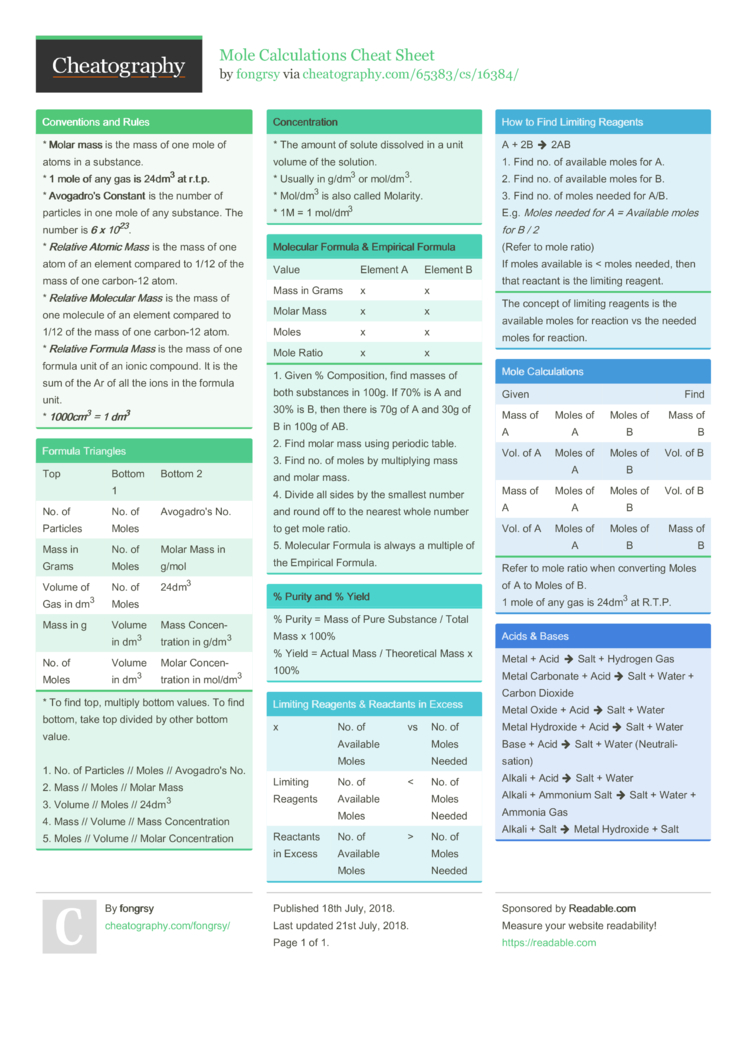# Mole Calculations Cheat Sheet by fongrsy

### Conven­tions and Rules

 * Molar mass is the mass of one mole of atoms in a substance. * 1 mole of any gas is 24dm3 at r.t.p. * Avog­adro's Constant is the number of particles in one mole of any substance. The number is 6 x 1023. * Rel­ative Atomic Mass is the mass of one atom of an element compared to 1/12 of the mass of one carbon-12 atom. * Rel­ative Molecular Mass is the mass of one molecule of an element compared to 1/12 of the mass of one carbon-12 atom. * Rel­ative Formula Mass is the mass of one formula unit of an ionic compound. It is the sum of the Ar of all the ions in the formula unit. * 100­0cm3 = 1 dm3

### Formula Triangles

 Top Bottom 1 Bottom 2 No. of Particles No. of Moles Avogadro's No. Mass in Grams No. of Moles Molar Mass in g/mol Volume of Gas in dm3 No. of Moles 24dm3 Mass in g Volume in dm3 Mass Concen­tration in g/dm3 No. of Moles Volume in dm3 Molar Concen­tration in mol/dm3
* To find top, multiply bottom values. To find bottom, take top divided by other bottom value.

1. No. of Particles // Moles // Avogadro's No.
2. Mass // Moles // Molar Mass
3. Volume // Moles // 24dm3
4. Mass // Volume // Mass Concen­tration
5. Moles // Volume // Molar Concen­tration

### Concen­tration

 * The amount of solute dissolved in a unit volume of the solution. * Usually in g/dm3 or mol/dm3. * Mol/dm3 is also called Molarity. * 1M = 1 mol/dm3

### Molecular Formula & Empirical Formula

 Value Element A Element B Mass in Grams x x Molar Mass x x Moles x x Mole Ratio x x
1. Given % Compos­ition, find masses of both substances in 100g. If 70% is A and 30% is B, then there is 70g of A and 30g of B in 100g of AB.
2. Find molar mass using periodic table.
3. Find no. of moles by multip­lying mass and molar mass.
4. Divide all sides by the smallest number and round off to the nearest whole number to get mole ratio.
5. Molecular Formula is always a multiple of the Empirical Formula.

### % Purity and % Yield

 % Purity = Mass of Pure Substance / Total Mass x 100% % Yield = Actual Mass / Theore­tical Mass x 100%

### Limiting Reagents & Reactants in Excess

 x No. of Available Moles vs No. of Moles Needed Limiting Reagents No. of Available Moles < No. of Moles Needed Reactants in Excess No. of Available Moles > No. of Moles Needed

### How to Find Limiting Reagents

 A + 2B  2AB 1. Find no. of available moles for A. 2. Find no. of available moles for B. 3. Find no. of moles needed for A/B. E.g. Moles needed for A = Available moles for B / 2 (Refer to mole ratio) If moles available is < moles needed, then that reactant is the limiting reagent.
The concept of limiting reagents is the available moles for reaction vs the needed moles for reaction.

### Mole Calcul­ations

 Given Find Mass of A Moles of A Moles of B Mass of B Vol. of A Moles of A Moles of B Vol. of B Mass of A Moles of A Moles of B Vol. of B Vol. of A Moles of A Moles of B Mass of B
Refer to mole ratio when converting Moles of A to Moles of B.
1 mole of any gas is 24dm3 at R.T.P.

### Acids & Bases

 Metal + Acid  Salt + Hydrogen Gas Metal Carbonate + Acid  Salt + Water + Carbon Dioxide Metal Oxide + Acid  Salt + Water Metal Hydroxide + Acid  Salt + Water Base + Acid  Salt + Water (Neutr­ali­sation) Alkali + Acid  Salt + Water Alkali + Ammonium Salt  Salt + Water + Ammonia Gas Alkali + Salt  Metal Hydroxide + Salt1 Page
//media.cheatography.com/storage/thumb/fongrsy_mole-calculations.750.jpg

PDF (recommended)